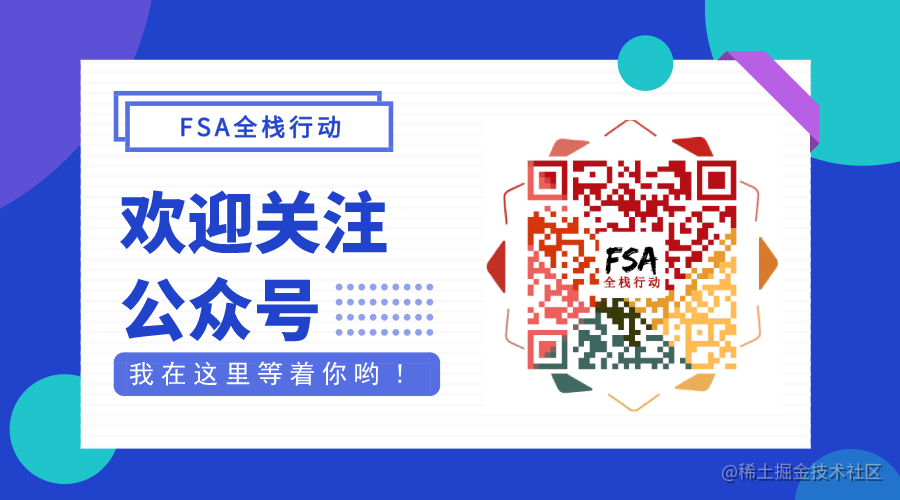# Kotlin - 函数与Lambda表达式

## 函数

``````fun sum(arg1: Int, arg2: Int): Int {
return arg1 + arg2
}

``````fun sum(arg1: Int, arg2: Int) = arg1 + arg2

### 默认返回值类型

``````fun main(args: Array<String>) {
...
}

``````fun main(args: Array<String>): Unit {
...
}

### 匿名函数

``````// 变量sum，它的值是一个函数
val sum = fun(arg1: Int, arg2: Int): Int {
return arg1 + arg2
}

fun main(args: Array<String>): Unit {
val result = sum(1, 2)
println(result) // 3
}

## Lambda 表达式

Lambda 表达式也是一种"匿名函数"，不过格式上会有些不同：

• {[参数列表] -> [函数体, 最后一行是返回值]}
``````val sum = { arg1: Int, arg2: Int ->
println("\$arg1 + \$arg2 = \${arg1 + arg2}")
arg1 + arg2
}

fun main(){
println(sum(1, 2)) // 3
}

Lambda 表达式的返回值是表达式中最后一行的结果。

### Lambda 的类型

Kotlin 中，不管是常量或是变量，都会有各自的类型，如：Human，那如果一个变量的值是 Lambda 表达式，那它的类型是什么呢？没错，就是 Lambda 类型：

• () -> Unit : 无参，返回值为 null
• (Int) -> Int : 传入整型，返回一个整型
• (String, (String)->String) -> Boolean : 传入字符串、Lambda 表达式，返回 Boolean
``````// lambda的类型：(Int,Int) -> Int
val sum = { arg1: Int, arg2: Int -> arg1 + arg2 }

// lambda的类型：() -> Unit
val printlnHello = {
println("Hello")
}

### Lambda 表达式的调用

• `()` 进行调用
• `invoke()` 调用
``````println(sum(1, 2)) // 3
println(sum.invoke(1, 2)) // 3

### Lambda 表达式的简化

• 函数参数调用时最后一个 Lambda 可以移出去
• 函数参数只有一个 Lambda，调用时小括号可省略
• Lambda 只有一个参数可默认为 it
• 入参、返回值与形参一致的函数可以用函数引用的方式作为实参传入

``````/**
* Performs the given [action] on each element.
*/
public inline fun <T> Array<out T>.forEach(action: (T) -> Unit): Unit {
for (element in this) action(element)
}

``````val args = arrayOf("1", "2", "3", "l", "q", "r")

// 使用 for-in 遍历数组并打印元素内容
for (arg in args) {
println(arg)
}

``````// Lambda完整写法
args.forEach({ it -> println(it) })

// Lambda简化写法
args.forEach({ println(it) })

// 重命名参数it，此时ele不可省略
args.forEach({ ele -> println(ele) })

Lambda 只有一个参数时可默认为 it，当然了，如果你不想使用 it 也是可以改名的，但如果改名了，就不能将其省略。除了省略默认参数 it，还有如下方法简化 Lambda 表达式：

``````// 函数参数调用时最后一个 Lambda 可以移出去
args.forEach(){ println(it) }

// 函数参数只有一个 Lambda，调用时小括号可省略
args.forEach{ println(it) }

### Lambda 表达式与函数引用

``````// action: (T) -> Unit
public inline fun <T> Array<out T>.forEach(action: (T) -> Unit): Unit {
for (element in this) action(element)
}

``````// println: (Any?) -> Unit
public actual inline fun println(message: Any?) {
System.out.println(message)
}

``````// 入参、返回值与形参一致的函数可以用函数引用的方式作为实参传入
args.forEach(::println)

### Lambda 表达式跳出 forEach

``````val args = arrayOf("1", "2", "3", "l", "q", "r")
for (arg in args) {
if (arg == "q") break
println(arg)
}
println("The End") // 1 2 3 l The End

``````fun main() {
val args = arrayOf("1", "2", "3", "l", "q", "r")
args.forEach {
if (it == "q") break // IDE报错：'break' and 'continue' are only allowed inside a loop
print("\$it ")
}
println("The End")
}

``````fun main() {
val args = arrayOf("1", "2", "3", "l", "q", "r")
args.forEach { // 1 2 3 l
if (it == "q") return
print("\$it ")
}
println("The End") // 不会被执行
}

``````fun main() {
val args = arrayOf("1", "2", "3", "l", "q", "r")
args.forEach {
if (it == "q") return@forEach
print("\$it ")
}
println("The End") // 1 2 3 l r The End
}

``````val args = arrayOf("1", "2", "3", "l", "q", "r")
args.takeWhile { it != "q" }.forEach { print("\$it ") }
println("The End")　// 1 2 3 l The End